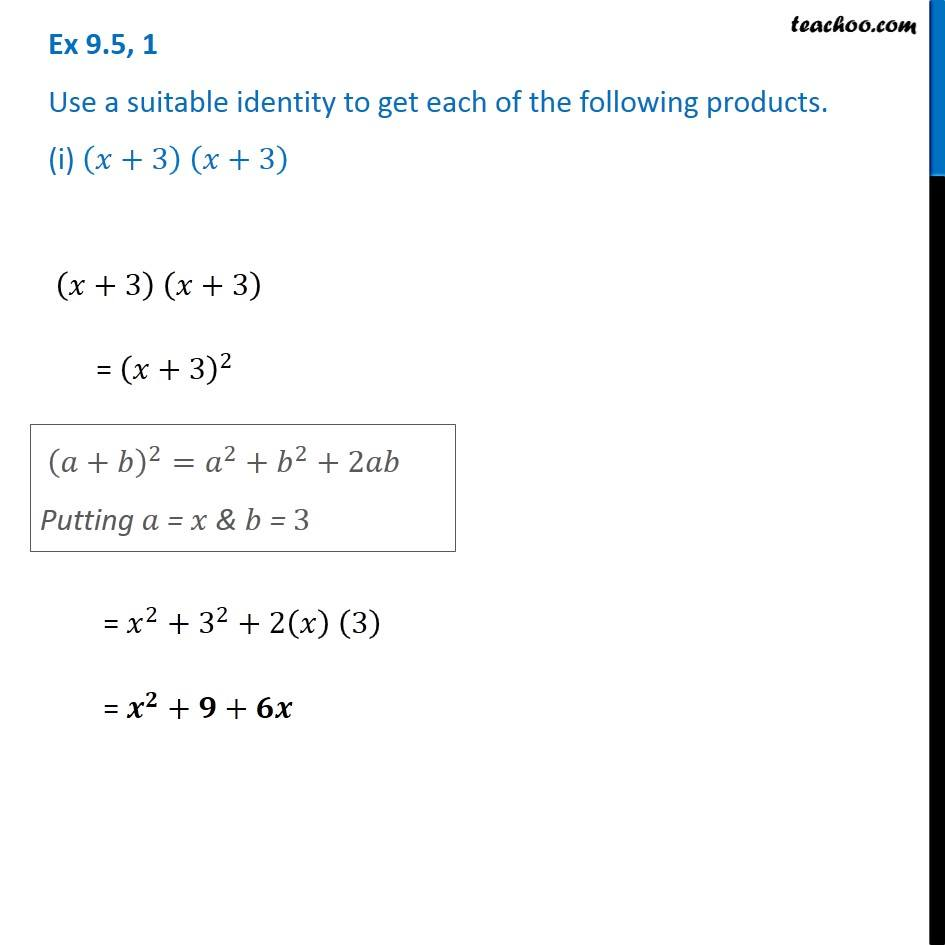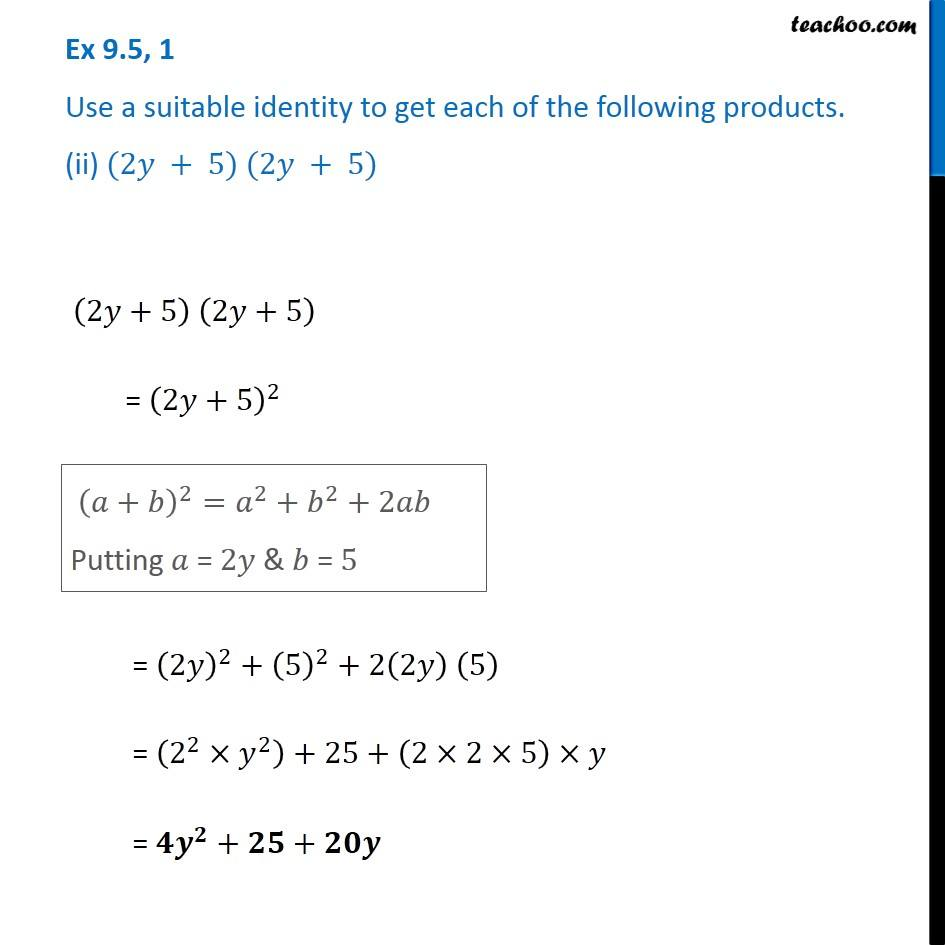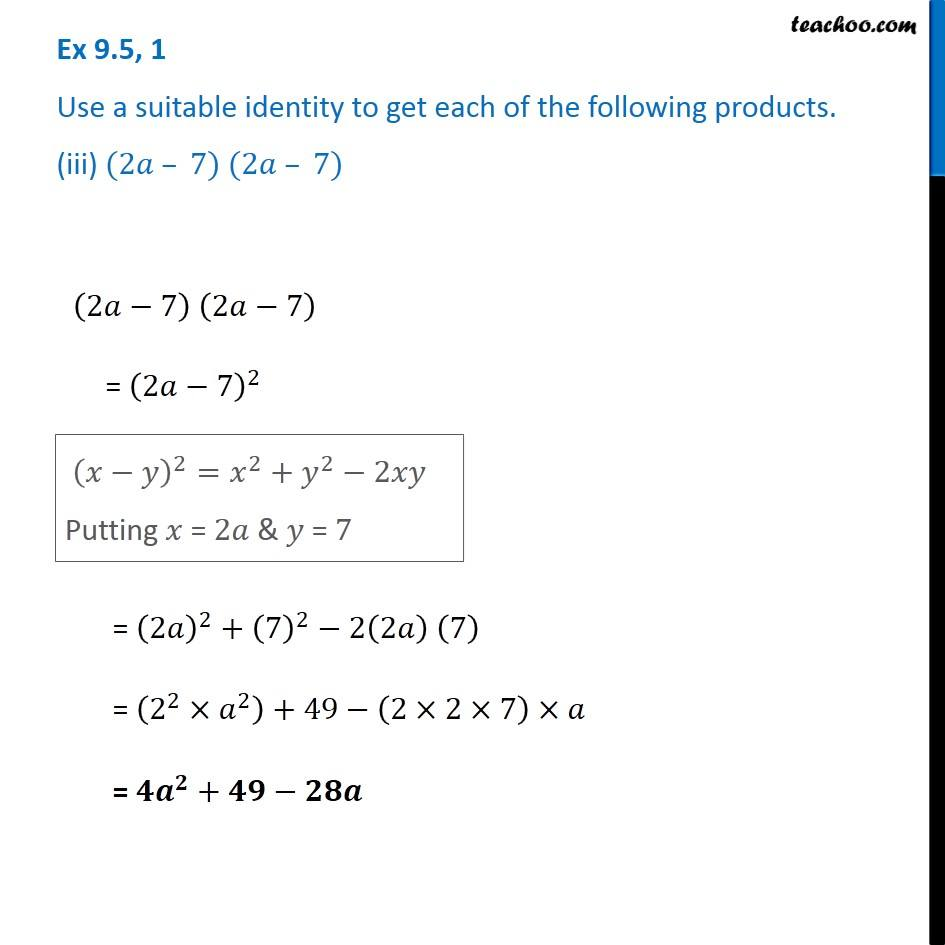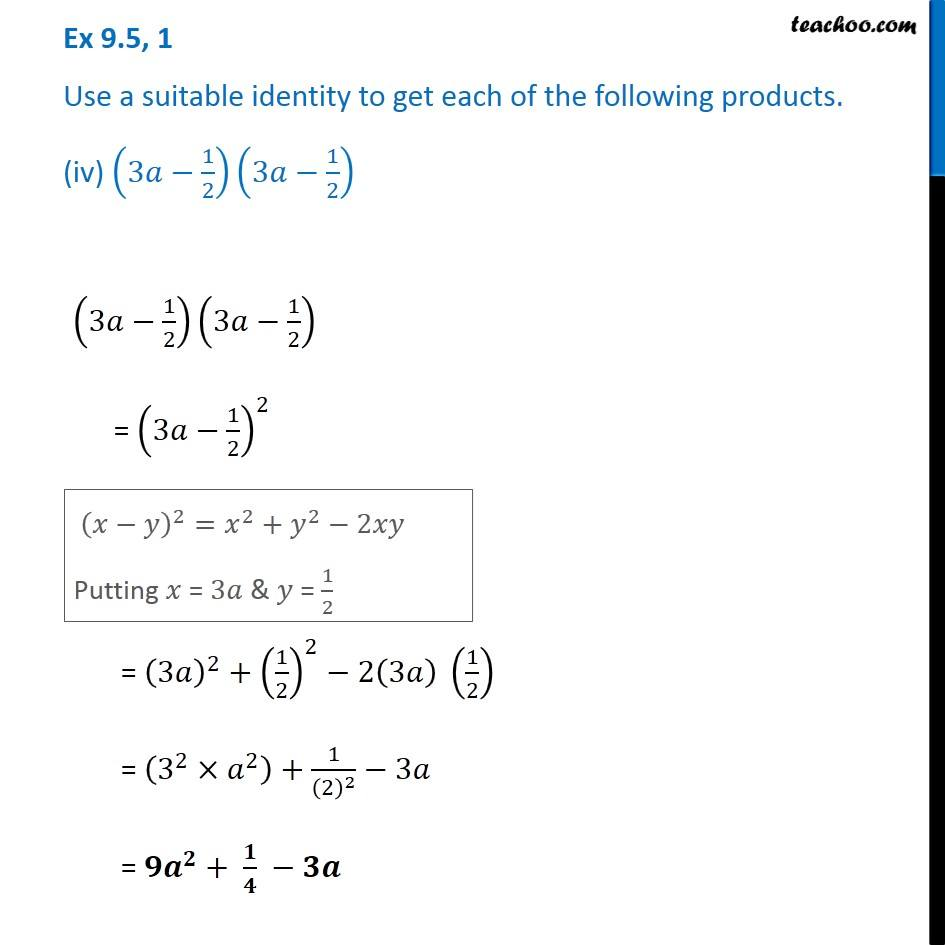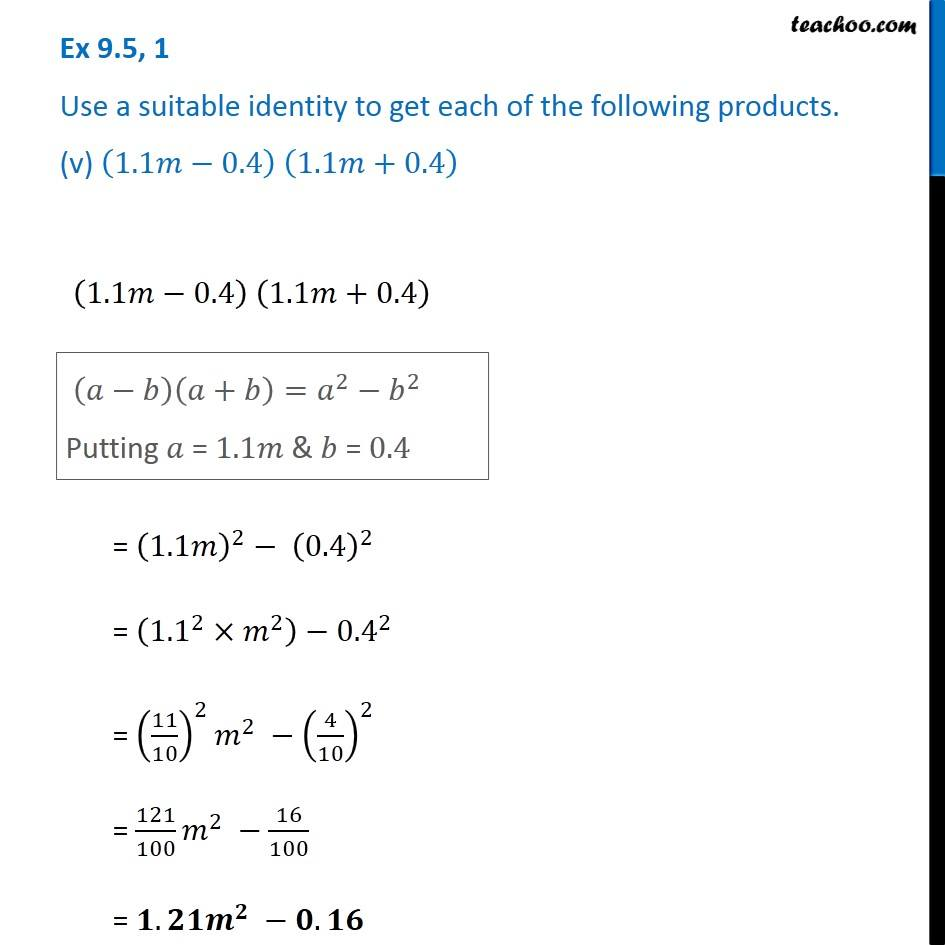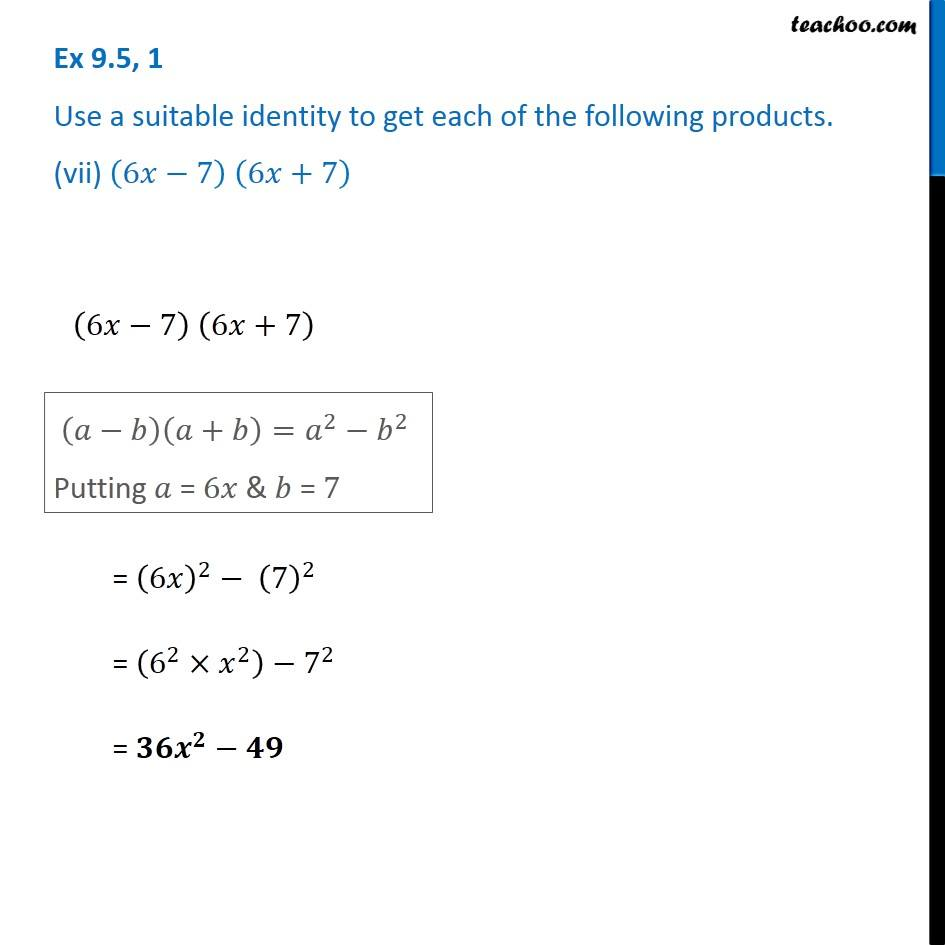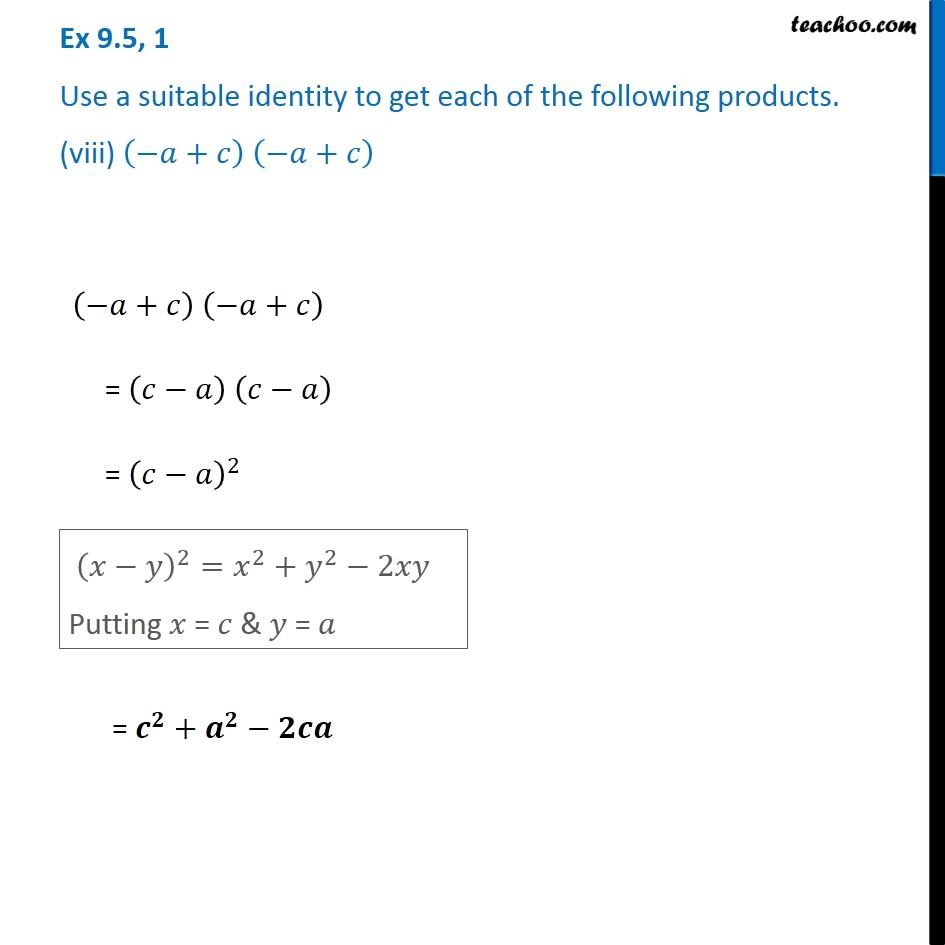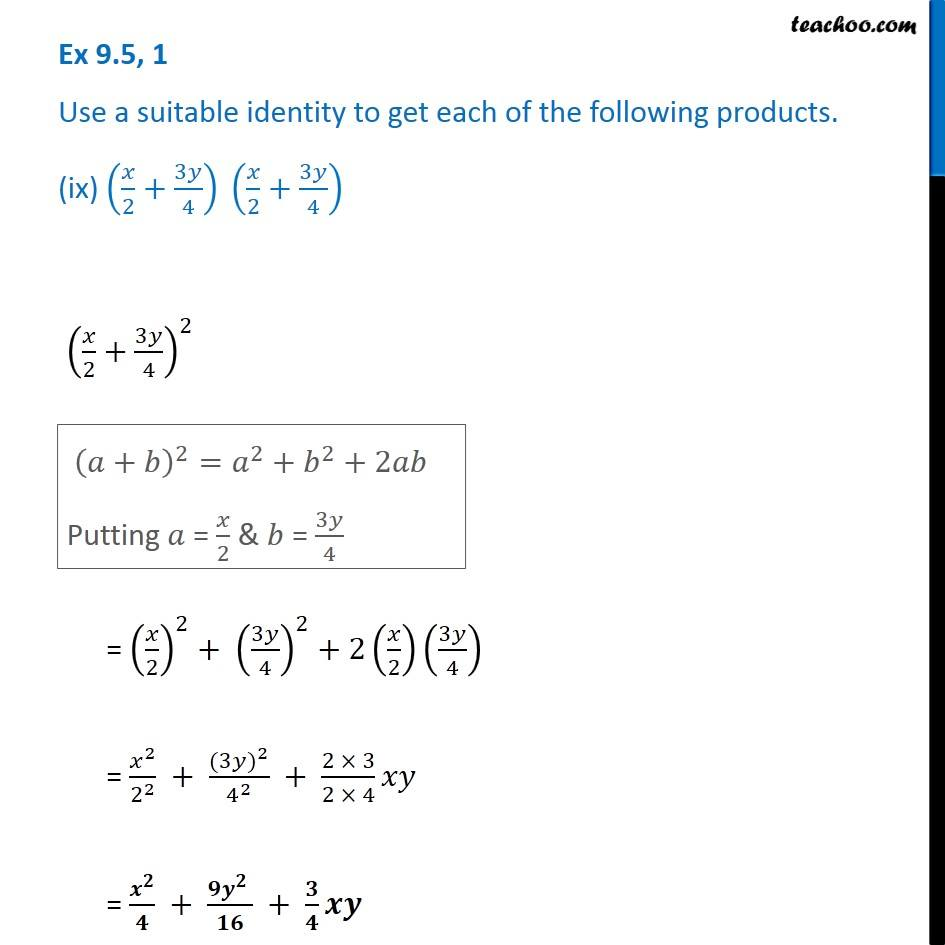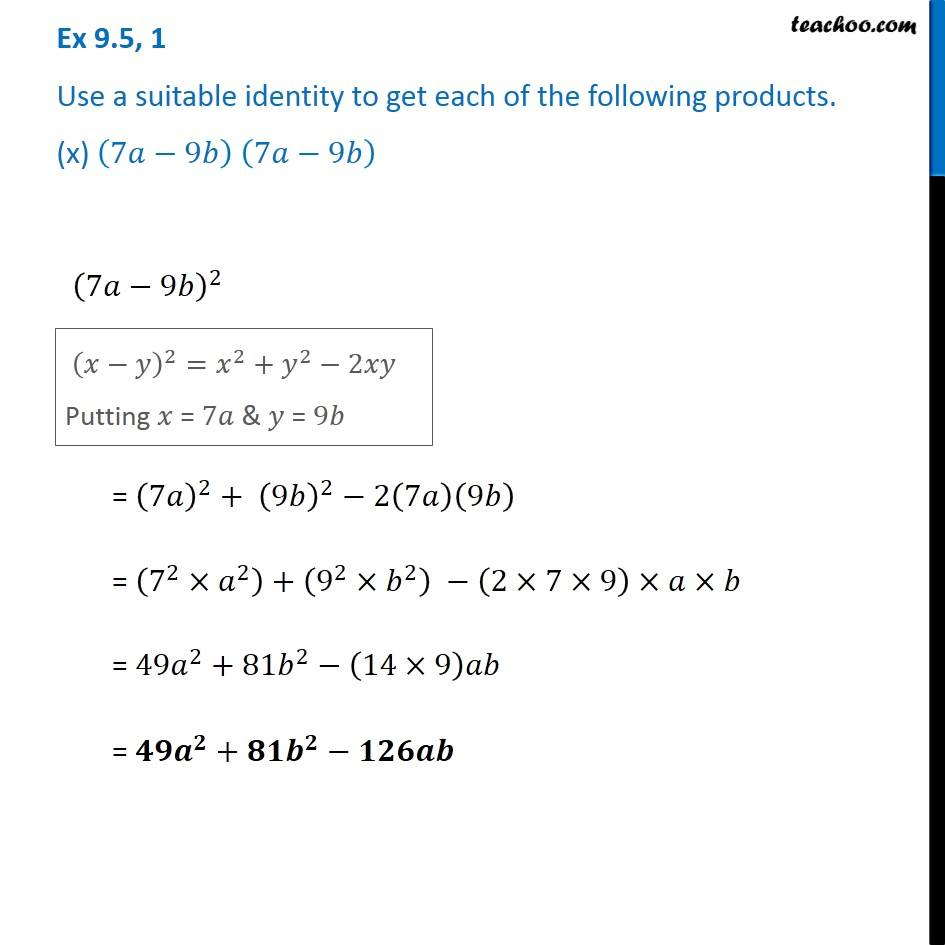1. Chapter 9 Class 8 Algebraic Expressions and Identities
2. Concept wise
3. Algebra Identities - Identity II

Transcript

Ex 9.5, 1 Use a suitable identity to get each of the following products. (i) (𝑥+3) (𝑥+3) (𝑥+3) (𝑥+3) = (𝑥+3)^2 (𝑎+𝑏)^2=𝑎^2+𝑏^2+2𝑎𝑏 Putting 𝑎 = 𝑥 & 𝑏 = 3 = 𝑥^2+3^2+2(𝑥) (3) = 𝒙^𝟐+𝟗+𝟔𝒙 Ex 9.5, 1 Use a suitable identity to get each of the following products. (ii) (2𝑦 + 5) (2𝑦 + 5) (2𝑦+5) (2𝑦+5) = (2𝑦+5)^2 (𝑎+𝑏)^2=𝑎^2+𝑏^2+2𝑎𝑏 Putting 𝑎 = 2𝑦 & 𝑏 = 5 (𝑎+𝑏)^2=𝑎^2+𝑏^2+2𝑎𝑏 Putting 𝑎 = 2𝑦 & 𝑏 = 5

Algebra Identities - Identity II